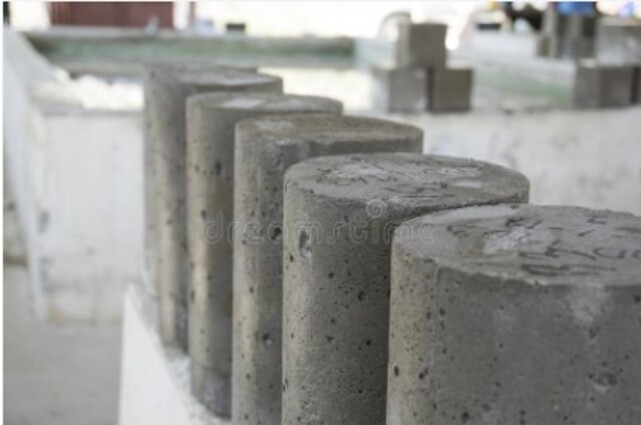# Compressive Strength of Concrete

Last Updated on July 25, 2022

Compressive strength of concrete is affected by many factors, including the water-cement ratio, cement strength, the grade of the concrete material, quality control during the manufacturing process which are explained below in the article.

## What is Strength in Civil Engineering?

A fundamental subject in civil engineering is strength of materials since it serves as the foundation for all other structural subjects such as structural analysis and structural design. Additionally, it has uses in fields such as geotechnical engineering.

Strength is an important property of materials, which is used by civil engineers when designing structures. The structure is prevented from failure if the load is kept within the strength limit. This shows that the maximum load must always be lower than the strength limit. Typically, this is accomplished by first figuring out the maximum load.

## What is Compressive Strength?

The capacity of a material or structure to carry loads on its surface without cracking or deflection is referred to as compressive strength. When a material is compressed, its size appears to shrink, while when it is tensioned, its size elongates.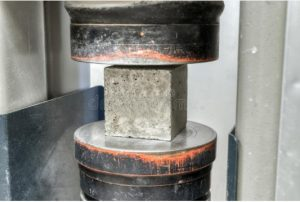Some materials crack as their compressive strength reaches its maximum, while others deform irreversibly.
Compressive strength is an important value in civil and structural engineering to consider when building structures. Engineers use the compressive strength of concrete as the most common performance evaluation when constructing buildings and other structures.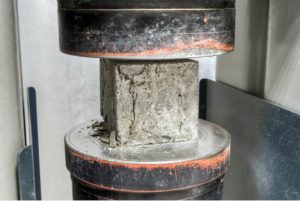Compressive strength is a compressive stress limit condition that causes material failure in the form of ductile failure (infinite theoretical yield) or brittle failure (rupture as the result of crack propagation, or sliding along a weak plane). Materials, elements and systems have all checked for compressive strength. The ultimate compressive strength of a material is described as the value of uniaxial compressive stress obtained when the material fails completely.

## What is Stress of a Material?

Stress is defined as the force of resistance per unit area provided by a body against deformation. The load or force is the external force acting on the body. The load is applied to the body, while stress is induced in the material. When the resistance given by the member against deformation and the applied load are equal, the loaded member remains in equilibrium.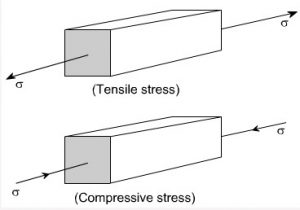σ=P/A

where σ = Stress (Pa),

P = External force or load (N),

and A = Cross-sectional area (m2).

## What is Strain of a Material?

Strain is a system’s response to applied stress.   When a material is subjected to a force, it generates stress, which causes the material to deform. The sum of deformation in the direction of the applied force divided by the original length of the material is known as engineering strain. This yields a unitless number, which is sometimes left in its natural form, such as inches per inch or meters per meter. To illustrate , the strain in a tensioned bar is the amount of elongation or change in length divided by the initial length.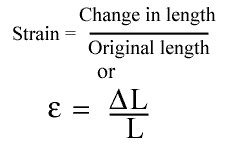If the stress is low, the material will only strain slightly and will return to its original size after the stress is released. This is known as elastic deformation since like elastic, it returns to its original state after being stressed. Elastic deformation in a material happens only when stresses are less than a critical stress known as the yield strength. If a material is loaded beyond its elastic limit, it will stay deformed even after the load is removed. This is known as plastic deformation.

## What is Elastic Modulus of Concrete?

The ratio of the applied stress to the resulting strain is known as the concrete modulus of elasticity (Ec). It demonstrates not only concrete’s ability to resist deformation due to applied stress, but also its stiffness. In other words, it represents concrete’s elastic deformation capacity. Concrete’s modulus of elasticity is affected by aggregate and mixture proportions. Concrete generally have an elastic modulus changing between 30 to 50 GPa.

The modulus of elasticity, which must be defined in the design of concrete structures, is highly important. In certain cases, such as the construction of pre-stressed concrete systems, the linear analysis of elements, which is based on elastic theory, is used to satisfy specifications of ultimate and serviceability limit states.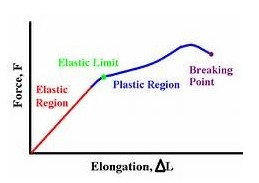To sum up, modulus of elasticity determines a material’s stiffness and is a good indicator of its strength. It’s the stress-to-strain ratio. Strain(∆L/L) is the deformation (change in shape) caused by stress, and stress is the deforming force acting per unit area (F/A).

## What is Compressive Strength Formula?

The load applied at the point of failure to the cross-section area of the face to which the load was applied is the compressive strength formula for any material.

Compressive Strength = Load (N)/ Cross-sectional Area(m2)

N/m2 = Pa    kPa=1000 Pa   MPa=1000 kPa        GPa=1000 MPa

## What is Compressive Strength of Concrete?

To ensure the required strength of the concrete during casting, the compressive strength of concrete is determined in batching plant laboratories by experiments. The strength of concrete is necessary to calculate the strength of the members and foundation of a structure. To determine the strength of concrete, concrete specimens are cast and checked under the action of compressive loads.

In brief, compressive strength is determined by dividing the failure load by the area of application of load, which is normally calculated after 28 days of curing. The proportioning of cement, coarse and fine aggregates, water, and multiple admixtures determines the strength of concrete. The water-to-cement ratio is the most important factor in determining concrete strength. The compressive strength increases as the water-cement ratio decreases.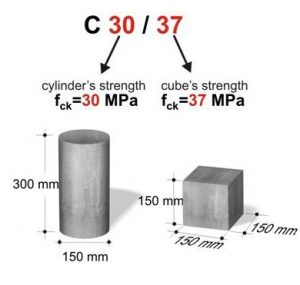When it comes to evaluating existing structures, compressive strength is an essential parameter to use. It’s used by structural engineers to model different structural properties such as tensile strength and to predict the flexural and shear ability of the elements. Other structural properties, such as ductility and stiffness, can also be described as functions of compressive strength.

## What are the main factors affecting compressive strength of concrete?

### 1. Quality of Materials

Concrete’s primary components are cement, aggregate, sand and water. The strength of the concrete is affected by the quality of each component. Therefore, all materials should meet the requirements for usage in concrete. Sand should not be salty, size of the aggregates are not too big and small and pozzolanic cement and clean water are preferred in concrete.

### 2. Water – Cement Ratio

The initial spacing between cement grains increases with the water/cement ratio, as does the volume of air voids that remain unfilled by hydration products.  If the water/cement ratio is decreased, the workability of the concrete decreases for a given cement amount. Less water, more cement, and decreased workability are the results of a lower water-to-cement ratio. However, if the workability goes too low, it becomes challenging to compact the concrete, which lowers its strength. If full compaction is accomplished, the strength at any age depends only on the water-cement ratio for a specific combination of ingredients and environmental factors.

### 3. Curing

Curing is one of the most important process of RCC construction which leads to strength development and durability in concrete. After pouring and finishing concrete, curing maintains the proper moisture and temperature at the depth and close to the surface for the development of strength. In other words, curing supplies concrete with enough water to complete the hydration process uninterrupted, which is crucial for the development of strength.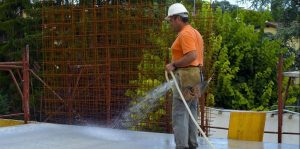### 4. Compaction of Concrete

Concrete is more dense when it is compacted because this procedure eliminates air voids from freshly poured concrete, making the concrete denser and compact. Concrete loses a lot of strength when air voids exist in it. Strength can be reduced by 30 to 40% in areas with 5% or more air voids.  Strength is greater in thoroughly compacted concrete than in inadequately compacted concrete.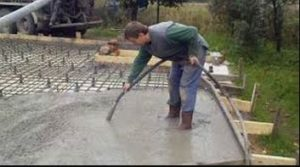### 5. Temperature

The rate of hydration process increases as the temperature goes up to a desired point, which allows it to quickly build strength. A thermal gradient is produced by sudden temperature changes, which causes concrete to crack and spall. As a result, at very high temperatures, compressive strength of concrete is decreased.

## How to measure the compressive strength of concrete?

Compressive strength test, mechanical test that determines the highest compressive load that a material can withstand before fracturing. A gradually applied load compresses the test specimen, which is typically in the shape of a square, prism, or cylinder, between the platens of a compression-testing unit.

The test sample can be a cylinder (15 cm x 30 cm ) or a cube (15 cm x 15cm x 15 cm) depending on the code used. ASTM C39, for example, specifies a common test procedure for determining the compressive strength of concrete cylinders.

1- Concrete is poured into the mold and properly compacted to reduce the air bubbles inside of it.

2- Moulds are removed after 24 hours, and test specimens are placed in water for curing.

3-After seven or 28 days of curing, these specimens are tested by using a compression testing machine.

5-To calculate the compressive strength of concrete, divide the failure load by the cross-sectional area of the specimen.

### Results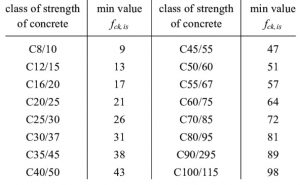Values are in MPa.

## What is the aim of compressive strength test?

Compression test measures fundamental variables including stress, strain, and deformation to determine a material’s behaviour or response to a compressive load. Compression test may give information about elastic limit, elastic modulus, compressive strength, yield strength, ultimate strength, among other parameters. It is possible to determine whether a material is suitable for specific applications or will fail under the defined stresses by considering these various parameters and the values associated with it

## What is Compression Testing Machine?

The compression testing machine is a widely used testing tool for determining a material’s compressive force or crush resistance, as well as its ability to recover after a given compressive force is applied and even maintained for a fixed period of time, by measuring fundamental variables such as stress, strain, and deformation. The compression test machine is available in a variety of models and capacities to satisfy the need for accurate and consistent testing of concrete samples.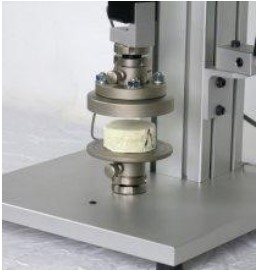Compressive strength of concrete has been explained in this article, hope you enjoyed it!